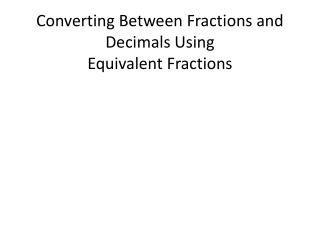DownloadDownload PresentationConverting Between Fractions and Decimals Using Equivalent Fractions

# Converting Between Fractions and Decimals Using Equivalent Fractions

Télécharger la présentation## Converting Between Fractions and Decimals Using Equivalent Fractions

- - - - - - - - - - - - - - - - - - - - - - - - - - - E N D - - - - - - - - - - - - - - - - - - - - - - - - - - -
##### Presentation Transcript

1. Question: What are some examples from the real world where decimals are used?

2. Question: What are some examples from the real world where fractions are used?

3. Question: Have you ever seen a recipe call for 2.7 cups of flour? Why or why not?

4. Question: How do you think people would react if a local gas station posted the price of gasoline as 3 3/7 dollars per gallon? Why?

5. What is the value of the number 2.25? How can this number be written as a fraction or mixed number? • b. Rewrite the fraction in its simplest form showing all steps that you use. • c. What is the value of the number 2.025? How can this number be written as a mixed number? • d. Rewrite the fraction in its simplest form showing all steps that you use

6. Use place value to convert each terminating decimal to a fraction. Then rewrite each fraction in its simplest form. • a. 0.218 • b. 0.16 • c. 2.72 • d. 0.0005